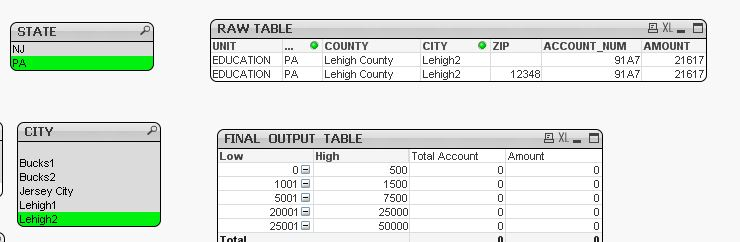Announcements
MAINTENANCE ALERT: Dec. 12th starting 9 AM CET. Community will be read-only. GET DETAILS
cancel
Showing results for
Did you mean:Creator II

## How to sum values based on selections at different levels

Hello,

Below is my raw table and final output table with bands (range). However, my expressions (Total Account & Amount) aren't working.

Attached is a sample qvw.

Based on what selection (State, County, City or Zip) is made, the Amount should fall in the corresponding range.
Selection can be one state or multiple states and so forth. I tried using set analysis but didn't quite get the correct result.

Any ideas how this can be handled? Thanks!1 Solution

Accepted SolutionsMVP

Try this

Sum({<ZIP = {"=If(GetSelectedCount(ZIP) > 0, Len(Trim(ZIP)) > 0, Len(Trim(ZIP)) = 0)"},

COUNTY = {"=If(GetSelectedCount(COUNTY) > 0 or GetSelectedCount(CITY) > 0 or GetSelectedCount(ZIP) > 0, Len(Trim(COUNTY)) > 0, Len(Trim(COUNTY)) = 0)"},

CITY = {"=If(GetSelectedCount(CITY) > 0 or GetSelectedCount(ZIP) > 0, Len(Trim(CITY)) > 0, Len(Trim(CITY)) = 0)"}>}AMOUNT) + Sum({1} 0)

6 RepliesMVP

What is the expected output for the data provided?Creator II
Author

Hi Sunny, please find the attached excel file for the expected output.MVP

This expression almost works... see if you have missed some data rows and it works for your original app

Sum({<ZIP = {"=If(GetSelectedCount(ZIP) > 0, Len(Trim(ZIP)) > 0, Len(Trim(ZIP)) = 0)"}, COUNTY = {"=If(GetSelectedCount(COUNTY) > 0, Len(Trim(COUNTY)) > 0, Len(Trim(COUNTY)) = 0)"}, CITY = {"=If(GetSelectedCount(CITY) > 0, Len(Trim(CITY)) > 0, Len(Trim(CITY)) = 0)"}>}AMOUNT) + Sum({1} 0)

Count(DISTINCT {<ZIP = {"=If(GetSelectedCount(ZIP) > 0, Len(Trim(ZIP)) > 0, Len(Trim(ZIP)) = 0)"}, COUNTY = {"=If(GetSelectedCount(COUNTY) > 0, Len(Trim(COUNTY)) > 0, Len(Trim(COUNTY)) = 0)"}, CITY = {"=If(GetSelectedCount(CITY) > 0, Len(Trim(CITY)) > 0, Len(Trim(CITY)) = 0)"}>}ACCOUNT_NUM) + Sum({1} 0)Creator II
AuthorHi Sunny, yes you are right - I think I missed some data rows. However, during my testing I found this expression didn't work in the above examples. Screen shots attached.

1) First image, when NJ state and Lehigh2 city is selected, the final table displays 0. Correct total account is 1 and amount is 21617
2) Second image, when NJ state and Lehigh1+Lehigh2 city is selected, the final table still displays 0. Correct total account is 2 and amount is 21617 + 1125 = 22742Creator II
Author

I meant state = PA (not NJ) in my prior response. Correction.MVP

Try this

Sum({<ZIP = {"=If(GetSelectedCount(ZIP) > 0, Len(Trim(ZIP)) > 0, Len(Trim(ZIP)) = 0)"},

COUNTY = {"=If(GetSelectedCount(COUNTY) > 0 or GetSelectedCount(CITY) > 0 or GetSelectedCount(ZIP) > 0, Len(Trim(COUNTY)) > 0, Len(Trim(COUNTY)) = 0)"},

CITY = {"=If(GetSelectedCount(CITY) > 0 or GetSelectedCount(ZIP) > 0, Len(Trim(CITY)) > 0, Len(Trim(CITY)) = 0)"}>}AMOUNT) + Sum({1} 0)Community Browser